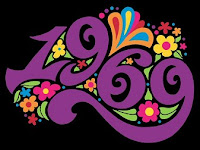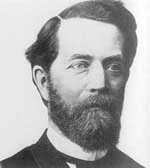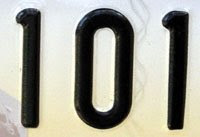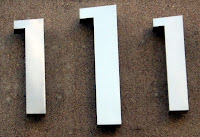## Friday, October 31, 2008

### 13

13 is a prime number.

13 has a representation as a sum of two squares: 13 = 22 + 32.

13 is the 7th Fibonacci number.

11 and 13 form a twin prime pair.

13
is a lucky number. To build the lucky number sequence, start with natural numbers. Delete every second number, leaving 1, 3, 5, 7, 9, 11, 13, 15, 17, 19, 21, . . . . The second number remaining is 3, so delete every third number, leaving 1, 3, 7, 9, 13, 15, 19, 21, . . . .The next number remaining is 7, so delete every 7th number, leaving 1, 3, 7, 9, 13, 15, 21, . . . . The next number remaining is 9, so delete every ninth number, and so on. The numbers that remain are lucky: 3, 7, 9, 13, 15, 21, 25, . . . .

132 = 169 and (reversing all digits) 312 = 961.

Three planes can cut a doughnut into a maximum of
13 parts.

13 is 111 in base 3.The fear of the number
13 is called triskaidekaphobia.

There are
13 tricks in each hand of bridge.

Source:
Number Gossip; Prime Curios!

## Thursday, October 30, 2008

### 13131

13131 = 32 x 1459.

13131 is an octahedral number.

13131 is palindromic in base 10 and in base 8 (31513).

13131 is a palindrome n such that 6n + 1 is also a palindrome: 78787.

13131 is the ninth-largest palindrome whose digit sum is 9.

13131 is a nonprime such that the sum of its digits equals the product of its digits: 1 + 3 + 1 + 3 + 1 = 9 = 1 x 3 x 1 x 3 x 1.13131 is the zip code for Parish, N.Y.

Source:
On-Line Encyclopedia of Integer Sequences

## Wednesday, October 29, 2008

### 1313

1313 = 13 x 101.

1313 is an evil number because it has an even number of 1s in its binary expansion: 10100100001.

1313 has two representations as a sum of two squares: 1313 = 172 + 322 = 232 + 282.

1313 is the hypotenuse of two primitive Pythagorean triples: 13132 = 2552 + 12882 = 7352 + 10882.

1313 is a divisor of 1006 - 1.The starburst galaxy NGC 1313 is known as the Topsy-Turvy Galaxy.The Italian poet Giovanni Boaccaccio was born in the year
1313.

Source:
ESO
Image: (c) ESO

## Tuesday, October 28, 2008

### 313

313 is a prime number, as is 10313 + 313.

313 has a representation as a sum of two squares: 313 = 122 + 132.

If 313 people are chosen at random, then the probability that at least five of them will share the same birthday is greater than 50%.

313 is 100111001 in base 2 (binary).

311 and 313 form a twin prime pair.

313 is the hypotenuse of a primitive Pythagorean triple: 3132 = 252 + 3122.In cartoons featuring Donald Duck, his car often sports the license plate number 313.

## Monday, October 27, 2008

### 1969

1969 = 11 x 179.

1969 is a composite number not ending in zero that yields a prime number when turned upside down: 6961 is prime.

1969
is the only known counterexample to a conjecture about modular Ackermann functions.

Now useful in computer science, the
Ackermann function was first studied by David Hilbert and Wilhelm Ackermann about 90 years ago. It grows faster than an exponential function or even a multiple exponential function.

The Ackermann function
A(x, y) is defined for integer x and y in the following way:
A(x, y) is y + 1 if x = 0; A(x – 1, 1) if y = 0; A(x – 1), A(x, y – 1) otherwise.

We can also restrict the range of the Ackermann function to a finite set, defining the mod-
n Ackermann function. For a given n, the numbers initially bounce around wildly as x increases, but nearly always stabilize to a certain value or an alternating pair of values.

According to Jerrold W. Grossman of Oakland University, the only value of
n less than 4,000,000 for which the standard mod-n Ackermann function does not stabilize is n = 1969. Why this is so remains a mystery.Neil Armstrong became the first man to step onto the moon's surface on July 20,
1969.

Source: Jon Froemke and Jerrold W. Grossman. 1993. A mod-n Ackermann function, or what’s so special about 1969? American Mathematical Monthly 100(February):180-183.

## Friday, October 24, 2008

### 1849

1849 = 432, a perfect square.

1849 is a perfect power in which neighboring digits are distinct.

1849 is a powerful number whose digit reversal is a semiprime: 9481 = 19 x 499.1849–1925) was born on April 25, 1849 (4/25/1849). Klein took particular delight in pointing out that each of the day (52), month (22), and year (432) is the square of a prime number.Source:
The MacTutor History of Mathematics Archive
Image:
Portrait Gallery

## Thursday, October 23, 2008

### 1221

1221 = 3 x 11 x 37.

1221 is a number that can be expressed as the product of numbers made of only one digit: 1221 = 11 x 111.

1221 is a divisor of 1003 - 1.

1221 is a number of the form n2 - 4, for n = 35.

1221 is 14341 (palindromic) in base 5. 1221 is 5353 in base 6, 3363 in base 7, and 633 in base 14,In the palindromic year 1221, the Maya of the Yucatán revolted against the rulers of Chichen Itza and Holy Roman Emperor Frederick II founded the University of Padua in Italy.

Source: Wikipedia

## Wednesday, October 22, 2008

### 234

234 = 2 x 32 x 13.

234 has a representation as a sum of two squares: 234 = 32 + 152.

234 is a solution to the postage stamp problem with four denominations and eight stamps.

There are 234 ways to stack 12 pennies in a line so that each penny lies on the table or on two pennies.234 is the atomic mass number of the rare, but naturally occurring, radioactive isotope uranium-234.

## Tuesday, October 21, 2008

### 292

292 = 22 x 73.

292 is 100100100 in base 2 (binary) and 444 in base 8.

292 has a representation as a sum of two squares: 292 = 62 + 162.There are 292 ways to make change for \$1 (U.S.), given the following coins: half dollar, quarter, dime, nickel, and penny.Source: Math Chat

## Friday, October 17, 2008

### 59

59 is the 17th smallest prime number.

59 is also a safe prime; that is, a prime of the form 2p + 1, where p is also a prime: 59 = 2 x 29 + 1.

An icosahedron has 59 stellations (including the icosahedron itself).

59 and 61 form a twin prime pair.M59 is a galaxy in the constellation Virgo.

Source: Wikipedia

## Thursday, October 16, 2008

### 49

49 is a perfect square: 49 = 72 . It is the first square whose digits are square.

49 is the smallest number with the property that it and its neighbors are squareful. In other words, each of these numbers contains at least one square in its factorization.

48 = 22 x 22 x 3
49 = 72
50 = 2 x 52

49 is the smallest composite number that is not divisible by any Fibonacci number greater than 1.

49 has a unique representation as a sum of three squares: 49 = 22 + 32 + 62.

49 is the smallest number with exactly eight representations as a sum of three distinct primes: 49 = 3 + 5 + 41 = 3 + 17 + 29 = 5 + 7 + 37 = 5 + 13 + 31 = 7 + 11 + 31 = 7 + 13 + 29 = 7 + 19 + 23 = 13 + 17 + 19.49 is the atomic number of the element indium.

Source: Number Gossip

## Wednesday, October 15, 2008

### 29

29 is the smallest multidigit prime number whose product of digits of its cube is also a cube: 293 = 24389 and 2 x 4 x 3 x 8 x 9 = 1728 = 123. 29 is also a Sophie Germain prime because 2 x 29 + 1 = 59 is prime.

29 has a representation as a sum of two squares: 29 = 22 + 52.

29 is the smallest prime equal to the sum of three consecutive squares: 22 + 32 + 42 = 29.

The
29th power of 2 is the largest power to 2 to have all different digits.

29 is the seventh Lucas number.

29 and 31 form a twin prime pair.

29 is the hypotenuse of a primitive Pythagorean triple: 292 = 202 + 212.The extra day in a leap year is February
29.

Source:
Number Gossip

## Tuesday, October 14, 2008

### 101

101 is a prime number. It is the smallest three-digit palindromic prime.

101 = 5! – 4! + 3! – 2! + 1!

101 is the sum of five consecutive primes: 13 + 17 + 19 + 23 + 29 = 101.

101 is the smallest odd prime that can be represented as the sum of a square and its reverse: 100 + 001 = 101.

A googol (10
100) contains 101 digits.John Nash's Princeton office number in the movie A Beautiful Mind was 101.

Source:
Prime Curios!

## Friday, October 10, 2008

### 1492

1492 = 22 x 373.

1492 has a representation as a sum of two squares: 1492 = 142 + 362.

1492 is a number whose square starts with three identical digits: 14922 = 2,226,064.

1492 is the least power of 2 that has exactly six consecutive zeros in its decimal representation.

The remainder when 17761492! is divided by 2000 is 1376. Remarkably, the remainder when 14921776! is divided by 2000 is also 1376.1492 was the year in which Christopher Columbus set out on his first voyage across the Atlantic Ocean to the Americas. It was a leap year (Julian calendar).

## Thursday, October 9, 2008

### 111

111 = 3 x 37.

111
is the smallest palindromic number such that the sum of its digits is one of its prime factors.

111 is a number that cannot be written as a sum of three squares.A six-by-six
magic square using the numbers 1 through 36 has a magic constant of 111.111 would be the magic constant for the smallest magic square composed only of prime numbers if one were counted as a prime.In J.R.R. Tolkien’s
The Lord of the Rings, Bilbo Baggins disappears from his eleventy-first (111th) birthday party and from the Shire.

Sources:
Number Gossip

## Wednesday, October 8, 2008

### 99

99 = 32 x 11.

99 is the largest number that is equal to the sum of its digits plus the product of its digits: 9 + 9 + 9 * 9.

99 is a Kaprekar number. Consider an n-digit number k. Square it and add the right n digits to the left n or n – 1 digits. If the resultant sum is k, then k is called a Kaprekar number.

992 = 9801
98 + 01 = 99

The first few Kaprekar numbers are 1, 9, 45, 55, 99, 297, 703, . . .

99 is 1100011 in base 2 (binary).99 is the maximum jersey number a player can wear in the National Football League.

Agent 99 was a character played by Barbara Feldon on the 1965-70 television show Get Smart and by Anne Hathaway in the 2008 movie version.

Sources: Number Gossip; Wolfram MathWorld

## Tuesday, October 7, 2008

### 248

248 = 23 x 31.

248 is a number n such that 6n - 1 and 6n + 1 are twin primes: 6 x 248 - 1 = 1487 and 6 x 248 + 1 = 1489.

248 is a number divisible by each of its digits.

If you're a mathematician doing a calculation and you get the answer 248, it means something completely different than if you get 247—because the number 248 shows up in all sorts of amazing places, while 247 is just dull.—John Baez, “My Favorite Numbers”

The exceptional Lie group
E8 has dimension 248.

In 1982, Michael Freedman used the
E8 lattice to construct an example of a topological 4-manifold that has no smooth structure.

In 2007, Garrett Lisi used
E8 in his “Exceptionally Simple Theory of Everything.”A picture of the E
8 root system.248 is one of the area codes for Oakland County, Mich.

Sources:
Wikipedia
Image:
American Institute of Mathematics